# positioning.inc

Various macros for positioning objects.

In the following examples, `S` is a sphere primitive with radius 0.5, and `C` is a cube of length 0.4.

`N_roots(N, R, Ob)`:

Constructs a union containing copies of object `Ob` at each `N`th root of unity in the xz-plane, at distance `R` from 0.N_roots(8,2,S)

`N_roots_SR(N, R, Ob, Sc, Rt)`:

Same as ```N_roots(N, R, Ob)```, with the `K`th copy of `Ob` scaled by `1+Sc*K` and rotated by `Rt*K` for each 0,...,`K`.N_roots_SR(8, 2, C, 0, 45*y)

`Row(Ob, Start, N, Space)`:

Constructs a union containing `N` copies of object `Ob` in a row, starting at point `Start` and spaced apart by vector `Space`.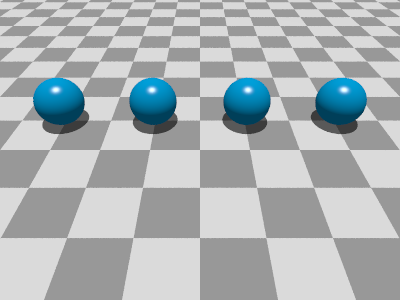Row(S, <-3, 0, 2>, 4, <2, 0, 0>)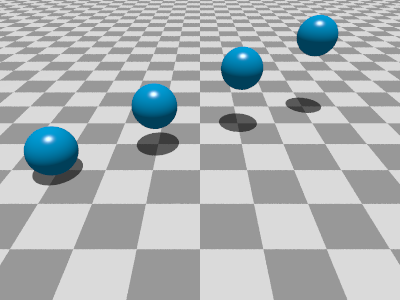Row(S, <-3, 0, 2>, 4, <2, 0.5, 1>)

`Row_SR(Ob, Start, N, Space, R, S)`:

Same as ```Row(Ob, Start, N, Space)``` but with the `K`th copy of `Ob` scaled by `1+S*K` and rotated by ```R*K for each 0,...,K.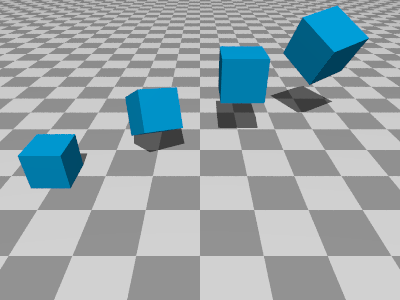Row_SR(C, <-3, 0, 2>, 4, <2, 0.5, 1>, <45,45,45>, 0.15) ```
```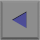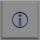```